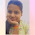When a beam of light of sufficiently high energy is made to fall on the surface of metal, electrons are ejected. This phenomenon is known as photoelectric effect and the electrons, thus ejected are known as photoelectrons.

Following observations were made in connection with this phenomenon.

I) The incident light should have a certain minimum frequency to eject electrons from a particular metal. This frequency is known as threshold frequency.

ii) The kinetic energy of the ejected electrons increases linearly with the frequency of the incident light and does not depend upon the intensity of the incident light.

iii) The number of photoelectrons ejected increase with the increase in the intensity of the incident light.

In 1905, Albert Einstein explained photoelectric effect on the basis of the quantum theory of radiation. According to Einstein, when a photon of energy hv strikes the surface of a metal, it gives its energy to an electron present on the metal surface. If the frequency v corresponding to the photons is equal to the threshold frequency, it gives its entire energy to the electron.
This energy enables the electron to come out of the surface by overcoming the attractive forces.

This equation is referred to as Einstein photo electric Equation. Einstein was awarded Nobel prize for physics in 1921 for explaining photoelectric effect and for his significant contribution to Mathematical physics.

1.1.